# Understanding Hardware Limitations

Please take a moment to review the Subject Interface Technical Specifications.

Important specifications particular to this guide:

 Stim Output Channels 16 per card Stim Output Voices 4 per card Stim Compliance Up to ± 15 V (load > 3 kΩ)± 5 mA per voice (load < 3 kΩ)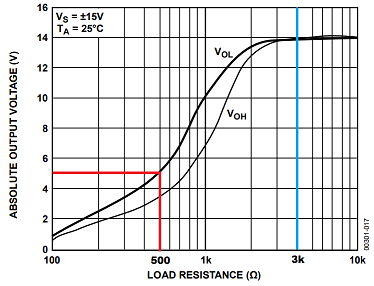The Subject Interface IVZ voltage compliance follows a curve that is load-dependent (figure to right). For impedance loads > 3 kΩ the output compliance per card is ± 15 V. At lower impedances, however, the output voltage compliance is reduced due to operation limits of the op amps in the IVZ.

The user should note that the current compliance limit of ± 5 mA for loads < 3 kΩ is strictly a consequence of Ohm's law (V = IR). Impedance loads > 3 kΩ would produce a voltage output larger than ± 15 V at a 5 mA current. Since the output request in this case is current and not voltage (current mode vs voltage mode), the voltage compliance curve shown does not apply, as you will see later.

## Output Impedance

Below are two diagrams that demonstrate what is occurring at a hardware level when a stimulus is output in either `Voltage Mode` or `Current Mode`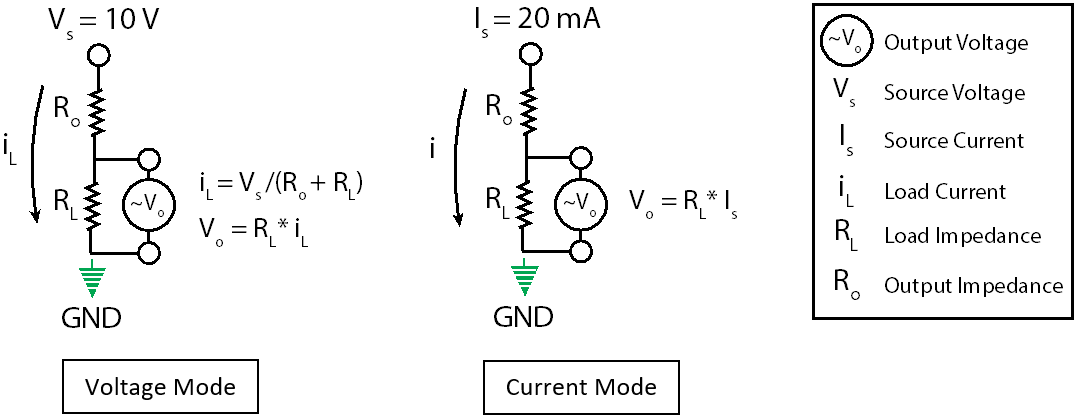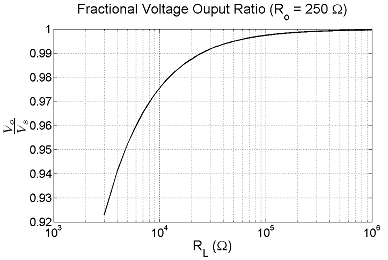As stated earlier, the absolute output voltage is limited by the impedance of the load resistor RL. Thus, the measured output voltage Vo when in Voltage Mode may not meet the requested voltage Vs. The issue is further compounded because the measured voltage Vo is affected by the output impedance Ro of the IZV. The voltage drop Vo across RL follows the scheme of a voltage divider, since some voltage is lost across Ro. Thus, your measured voltage will always be less than your requested voltage Vs for all impedance loads < 3 kΩ or > 3 kΩ, even if your requested voltage is below the compliance limit (see Fractional Voltage graph for expected loss and the example `Voltage Mode` oscilloscope output below).

In `Current Mode`, the requested current Is flows across both resistors equally. Thus, the measured Vo is not affected by Ro (see example `Current Mode` oscilloscope output below).

## Using < 3kΩ Resistance Loads

Below are oscilloscope images that show the measured voltage output across a 500 Ω resistor load (Ro). The test was performed in `Voltage Mode` and `Current Mode` to compare the compliance limits for a targeted 10 V output. The voltage division for each graph is 5 V.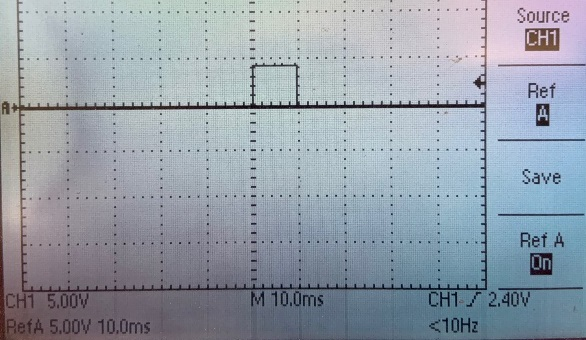Voltage Mode: RL = 500 Ω load, Vs = 10 V requested

The measured voltage is slightly less than the compliance maximum of ~ 5V for the 500 Ω load resistance due to Ro.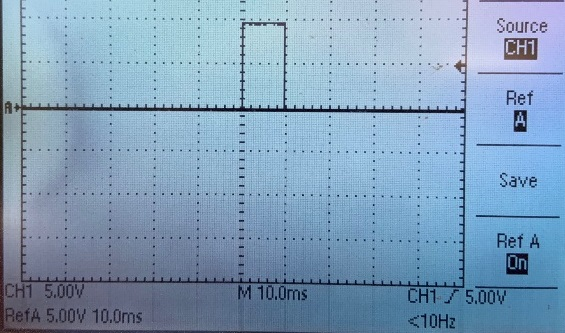Current Mode: Ro = 500 Ω load, Is = 20 mA requested

Current Doubling: By Four was active to achieve`{5 mA*4 Voices}` current compliance maximum `V = {I*R}; 10 V = {20mA*500 Ω}` to mimic the 10 V request test from `Voltage Mode` The measured voltage is 10 V because we used `Current Mode`.